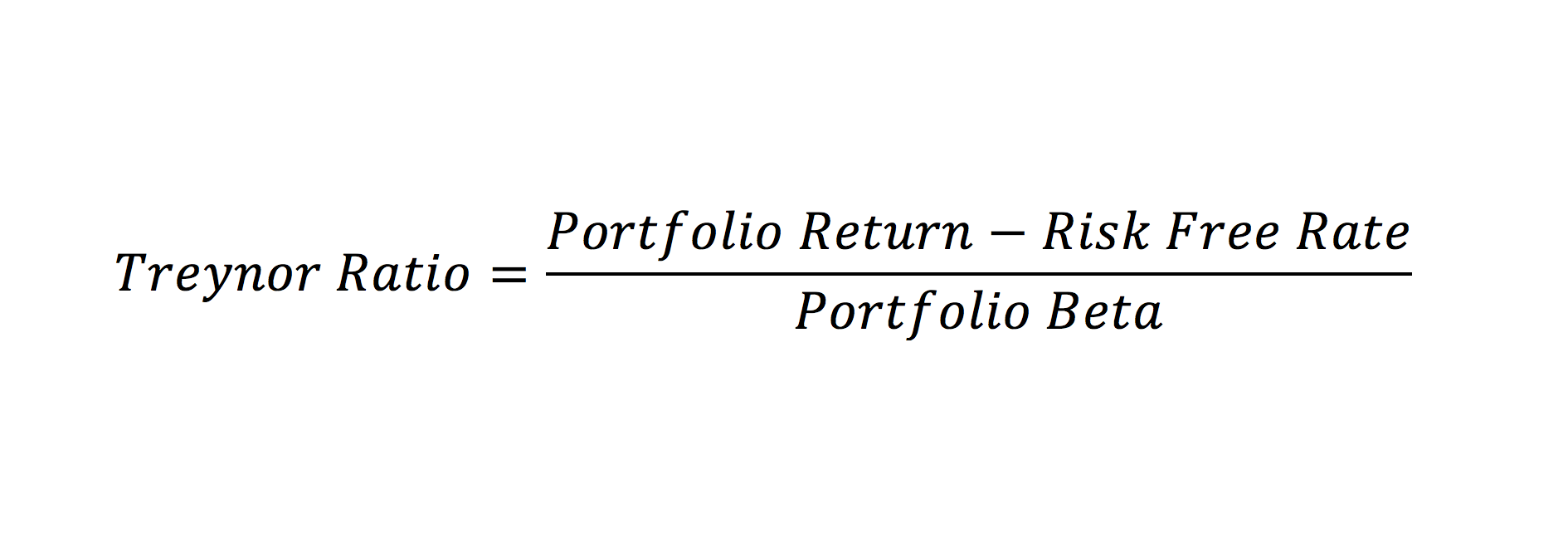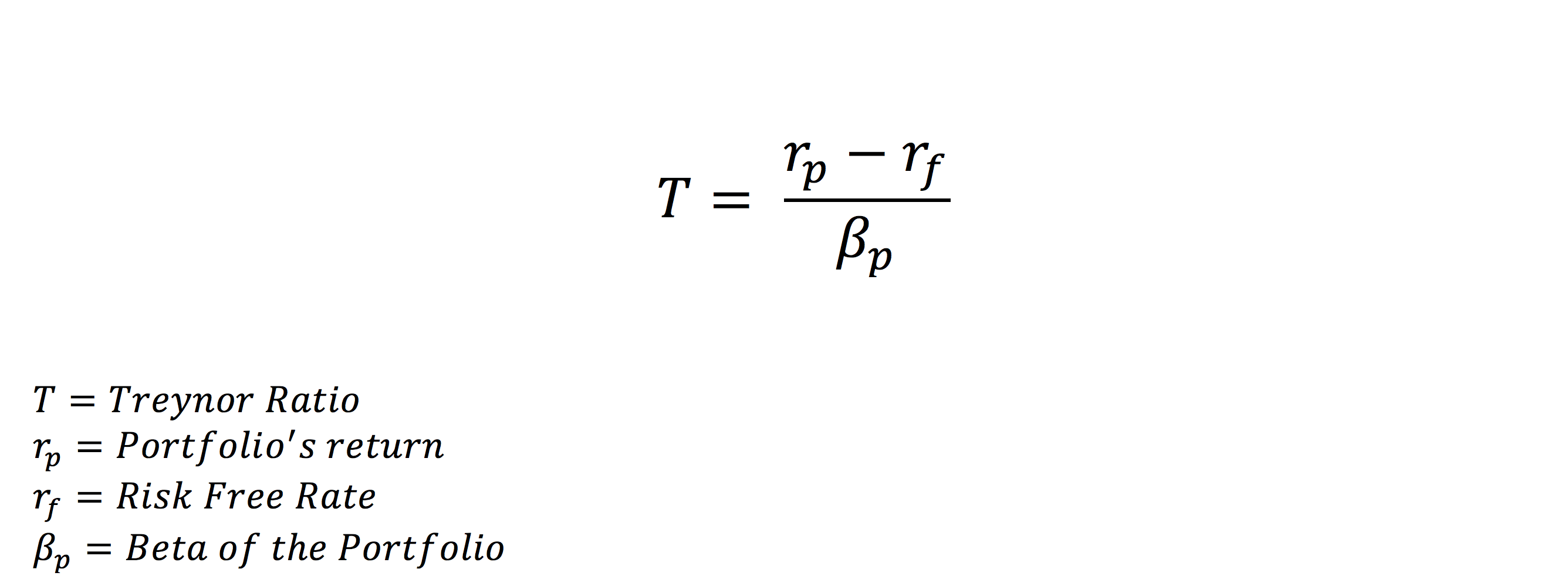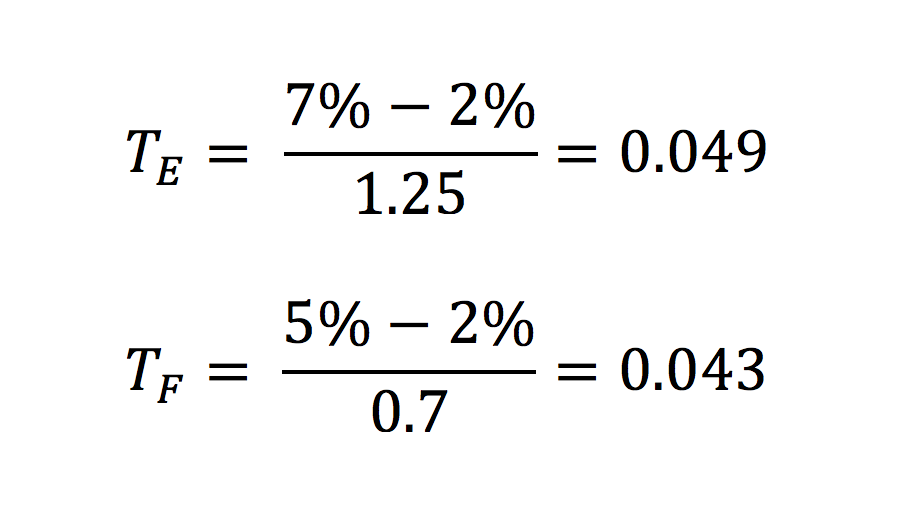# Treynor Ratio

A Risk-Adjusted Return Ratio

## What is the Treynor Ratio?

The Treynor Ratio is a portfolio performance measure that adjusts for systematic risk. In contrast to the Sharpe Ratio, which adjusts return with the standard deviation of the portfolio, the Treynor Ratio uses the Portfolio Beta, which is a measure of systematic risk.

These ratios are concerned with the risk and return performance of a portfolio and are a quotient of return divided by risk. The Treynor Ratio is named for Jack Treynor, an American economist known as one of the developers of the Capital Asset Pricing Model.### Treynor Ratio Formula

From the formula below, you can see that the ratio is concerned with both the return of the portfolio and its systematic risk. From a purely mathematical perspective, the formula represents the amount of excess return from the risk-free rate per unit of systematic risk. Like the Sharpe Ratio, it is a Return/Risk Ratio.The Treynor Ratio measures portfolio performance and is part of the Capital Asset Pricing Model. To read more about how to calculate Beta, click here.

### Treynor Ratio Example

Suppose you are comparing two portfolios, an Equity Portfolio and a Fixed Income Portfolio. You’ve done extensive research on both portfolios and can’t decide which one is a better investment. You decide to use the Treynor Ratio to help you select the best portfolio investment.

The Equity Portfolio’s total return is 7%, and the Fixed Income Portfolio’s total return is 5%. As a proxy for the risk-free rate, we use the return on U.S Treasury Bills – 2%. Assume that the Beta of the Equity Portfolio is 1.25, and the Fixed Income Portfolio’s Beta is 0.7. From the following information, we compute the Treynor Ratio of each portfolio.From the result above, we see that the Treynor Ratio of the Equity Portfolio is slightly higher. Thus, we can deduce that it is a more suitable portfolio to invest in. A higher Treynor ratio indicates a more favorable risk/return scenario. Keep in mind that Treynor Ratio values are based on past performance that may not be repeated in future performance.

As a financial analyst, it is important to not rely on a single ratio for your investment decisions. Other financial metrics should be considered before making a final decision.

### When using the Treynor Ratio, keep in mind:

• For negative values of Beta, the Ratio does not give meaningful values.
• When comparing two portfolios, the Ratio does not indicate the significance of the difference of the values, as they are ordinal. For example, a Treynor Ratio of 0.5 is better than one of 0.25, but not necessarily twice as good.
• The numerator is the excess return to the risk-free rate. The denominator is the Beta of the portfolio, or, in other words, a measure of its systematic risk.

### More Resources

Thank you for reading CFI’s explanation of the Treynor Ratio. CFI offers the Financial Modeling & Valuation Analyst (FMVA)™ certification program for those looking to take their careers to the next level. To keep learning and advancing your career, the following resources will be helpful:

• Capital Asset Pricing Model (CAPM)
• Internal Rate of Return (IRR)
• Treasury Bills (T-Bills)
• Financial Modeling Guide

### Financial Analyst Training

Get world-class financial training with CFI’s online certified financial analyst training program!

Gain the confidence you need to move up the ladder in a high powered corporate finance career path.

Learn financial modeling and valuation in Excel the easy way, with step-by-step training.